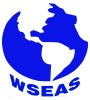Conference TopicsContributions related, but not limited, to the following topics of interest are welcome:

Analysis
Nonlinear Analysis
Algebra
Topology
Algebraic Topology
Lie Groups and Lie Algebras
C* Algebras
Functional Analysis
Geometry
Differential Geometry
Algebraic Geometry
Complex Analysis
Set Theory
Operators' Theory
Ordinary Differential Equations And Chaos
Partial Differential Equations
Inverse Problems
Banach Spaces
Convex Analysis
Ergodic Theory
Ergodic Theory and Symbolic Dynamics
Graph Theory
Manifolds
Modern Group Theory
Rings & Modules & Representations
Fields and Galois Theory
Algebraic K-Theory
Commutative Algebra
Harmonic Analysis
Measure Theory
Dynamical Systems
Topological Algebras
Mathematical Logic
Algebraic Number Theory
Analytic Number Theory
Probability & Statistics
Combinatorics
Combinatorics and Discrete Geometry
Module and Representation Theory
Non-Smooth Analysis
Mathematical Physics
Theoretical Topics in Numerical Analysis
Optimization and Variational Analysis
Theoretical Game Theory
Theoretical Fluid Dynamics
Theoretical Foundations of Control
Algorithms and Complexity
Others

## Bulletin Board

Currently:

The Conference Program is online.

The Conference Guide is online.